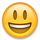# Intersection points of a DEdge and Region?

Hi,
Does anybody know a way using the Python API to find the intersection points of a DEdge with polygons in a Region? The intersection points should not be rounded to integers for my purpose.
It seems to be easy, but I just failed to find something.
Thanks!

• Hi tok,

you mean, the intersection with the polygon outline?

If you want good performance, there is no real option currently. If you're happy with Python performance, you could iterate over the Polygon's edges and use Edge#intersect:

``````dbu = 0.001  # should use layout.dbu

region = pya.Region()
region.insert(pya.Box(0, 0, 1000, 2000))

edge = pya.DEdge(-0.1, 0.5, 0.5, 0.5)

dbu_trans = pya.CplxTrans(dbu)

intersection_points = []

for poly in region.each():
for pe in poly.transformed(dbu_trans).each_edge():
if pe.intersect(edge):
intersection_points.append(pe.intersection_point(edge))

print(repr(intersection_points))
``````

Matthias

• Thanks for answering! Yes, this is the way I'm doing it now. As for now I'm only looking for intersections with a rectangle, so this solution is good enough. I thought that at the core of the boolean operations there must be something like this but I guess it is just not accessible by the API.

• Yes, internally there is code for this.

Basically it's even available when you perform a boolean "AND" between two edge sets - one with the polygon's edges and one with the single edge. Right now, this feature is intended for coincident edge selection, not for intersections.

But generalizing to "point-like" edges as output for intersections should be possible.

Matthias

• Ok, thanks! Yes, I think this would make KLayout an even more general geometry library.• I have created a ticket here: https://github.com/KLayout/klayout/issues/new

However, "DEdge" is difficult as there is no database unit in the general case. But "Edge" should be possible.

Matthias